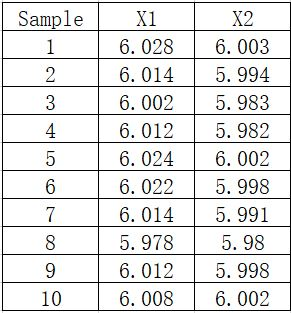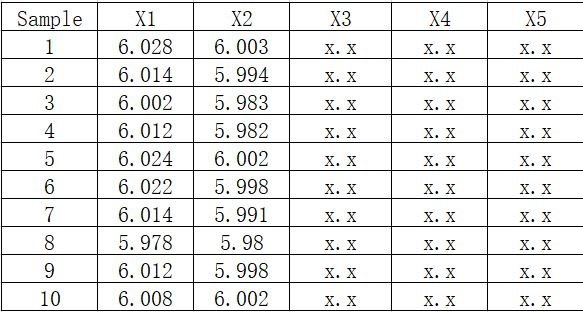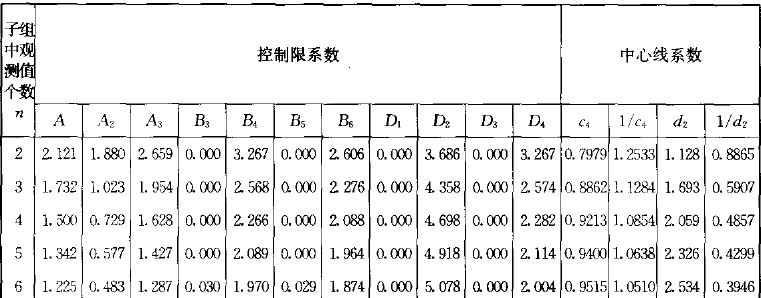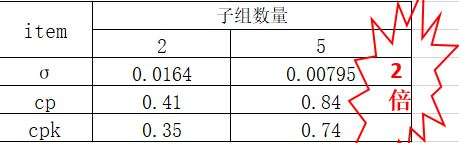## 子组数量大小对过程能力指数cp/cpk的影响###### ding我说的不一定对，望独立思考

0 人点赞了该文章 · 595 浏览σ=R/d2=0.0185/1.128=0.0164

cp=T/6*σ=0.04/6*0.0164=0.41

cpk=(usl-X)/3*σ=0.35σ=R/d2=0.0185/2.326=0.00795

cp=T/6*σ=0.04/6*0.00795=0.84

cpk=(usl-X)/3*σ=0.74###### 免责声明: